#### 相似文章# 神经网络：从理论到实践

MetaTrader 5示例 | 20 三月 2014, 06:1510 4784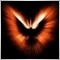### 神经网络的概念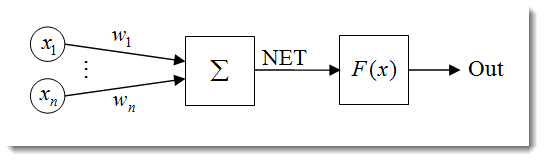1. 输入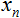2. 权重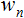3. 传递函数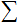和净输入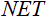4. 激活函数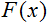5. 输出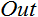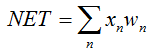在这里是神经元的净输入。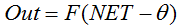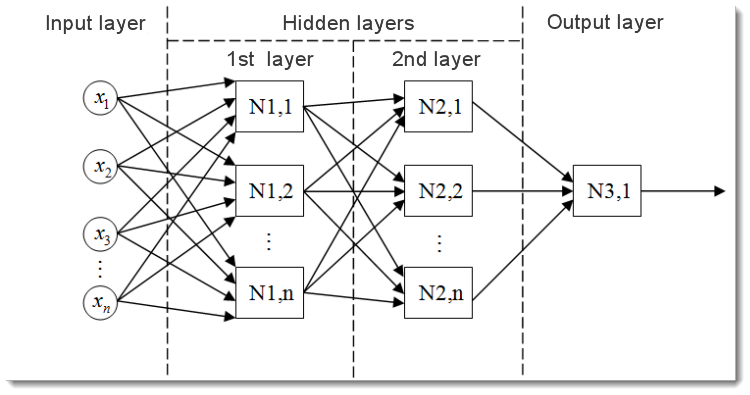• 输入层，用于将数据分配到网络，并且不执行任何计算。此层的输出将信号传递到下一层（隐藏层或输出层）的输入；
• 输出层，通常包含一个神经元（某些情况下不止一个），生成整个神经网络的输出。此信号是 EA 将来的控制逻辑的基础；
• 隐藏层，是标准神经元层，将信号从输入层传递到输出层。其输入是上一层的输出，而其输出用作下一层的输入。

### 输入数据正态化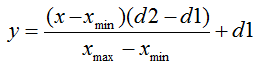•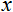- 要正态的值；
•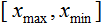- х 值的范围；
•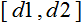- 要将 x 的值减小到的范围。

```double d1=0.0;
double d2=1.0;
double x_min=iMA_buf[ArrayMinimum(iMA_buf)];
double x_max=iMA_buf[ArrayMaximum(iMA_buf)];
for(int i=0;i<ArraySize(iMA_buf);i++)
{
inputs[i]=(((iMA_buf[i]-x_min)*(d2-d1))/(x_max-x_min))+d1;
}```

### 激活函数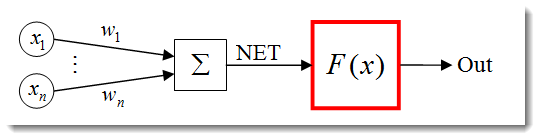•是激活函数；
•是计算神经元输出的第一阶段获得的加权和；
•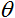是激活函数的阈值。它仅用于硬阈值函数，在其他函数中等于零。

1. 单位阶梯函数硬阈值函数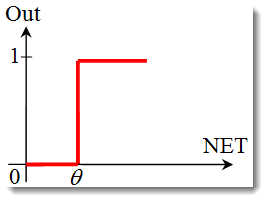用以下公式描述该函数：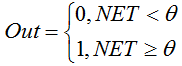如果加权和小于指定值，则激活函数返回 0。如果加权和大于指定值，则激活函数返回 1。

2. S 形函数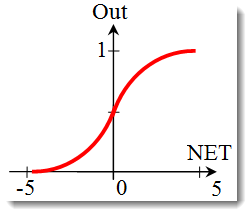描述 S 函数的公式如下：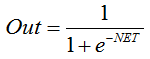它通常在多层神经网络以及具有连续信号的其它网络中使用。函数光滑度和连续性是非常有用的属性。

3. 双曲正切函数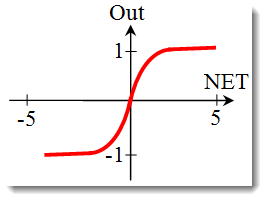公式：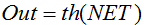or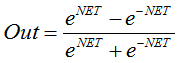它通常也在具有连续信号的网络中使用。可以返回负值是它的特点。

### 更改激活函数的形状```for(int n=0; n<10; n++)
{
NET+=Xn*Wn;
}```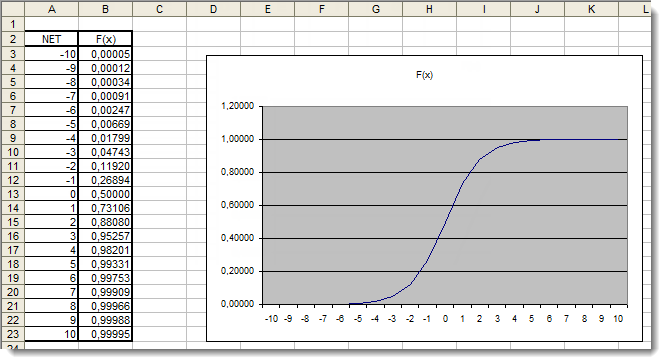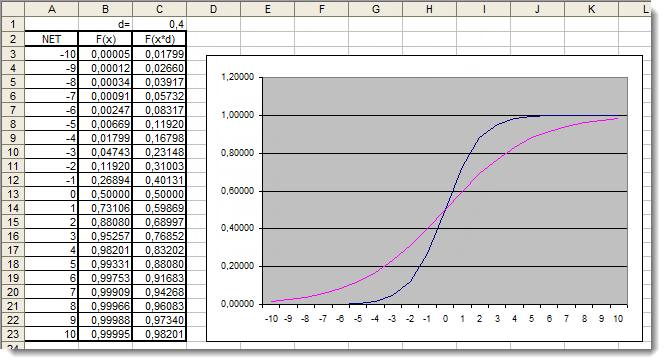```for(int n=0; n<10; n++)
{
NET+=Xn*Wn;
}
NET*=0.4;```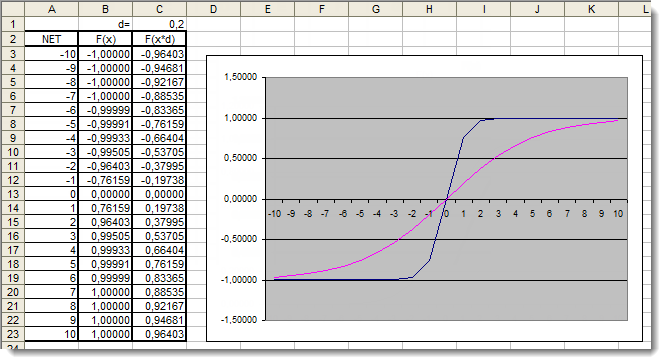```for(int n=0;n<10;n++)
{
NET+=Xn*Wn;
}
NET*=0.2;```

### 应用```double NET;
double x;
double w;
int OnInit()
{
x=0.1; // set the input value х1
x=0.8; // set the input value х2
x=0.5; // set the input value х3

w=0.5; // set the weight value w1
w=0.6; // set the weight value w2
w=0.3; // set the weight value w3

for(int n=0;n<3;n++)
{
NET+=x[n]*w[n]; // add the weighted net input values together
}
}```

1. 我们以声明一个用于存储神经元净输入的变量和两个数组：输入和权重开始；
2. 一开始就在所有函数的外部声明这些变量，以让它们具有全局作用范围（从而能够在程序中的任何地方访问）；
3. OnInit() 初始化函数中（实际上可以是任何其它函数），我们可以填写输入数组和权重数组；
4. 接着是求和循环，n<3，因为我们只有三个输入和三个对应的权重；
5. 再接着，我们将加权输入值加在一起，并将它们存储在变量中。

```double Out;
if(NET>=x) Out=1;
else Out=0;```

S 形函数

`double Out = 1/(1+exp(-NET));`

`double Out = (exp(NET)-exp(-NET))/(exp(NET)+exp(-NET));`

### 把所有内容串起来

```//+------------------------------------------------------------------+
//|                                                neuro-example.mq5 |
//|                        Copyright 2012, MetaQuotes Software Corp. |
//|                                              http://www.mql5.com |
//+------------------------------------------------------------------+
#property version   "1.00"
//+------------------------------------------------------------------+
//| Expert initialization function                                   |
//+------------------------------------------------------------------+
#include <Trade\PositionInfo.mqh> //include the library for obtaining information on positions

//--- weight values
input double w0=0.5;
input double w1=0.5;
input double w2=0.5;
input double w3=0.5;
input double w4=0.5;
input double w5=0.5;
input double w6=0.5;
input double w7=0.5;
input double w8=0.5;
input double w9=0.5;

int               iRSI_handle;  // variable for storing the indicator handle
double            iRSI_buf[];   // dynamic array for storing indicator values

double            inputs;   // array for storing inputs
double            weight;   // array for storing weights

double            out;          // variable for storing the output of the neuron

string            my_symbol;    // variable for storing the symbol
ENUM_TIMEFRAMES   my_timeframe; // variable for storing the time frame
double            lot_size;     // variable for storing the minimum lot size of the transaction to be performed

CPositionInfo     m_Position;   // entity for obtaining information on positions
//+------------------------------------------------------------------+
//|                                                                  |
//+------------------------------------------------------------------+
int OnInit()
{
//--- save the current chart symbol for further operation of the EA on this very symbol
my_symbol=Symbol();
//--- save the current time frame of the chart for further operation of the EA on this very time frame
my_timeframe=PERIOD_CURRENT;
//--- save the minimum lot of the transaction to be performed
lot_size=SymbolInfoDouble(my_symbol,SYMBOL_VOLUME_MIN);
//--- apply the indicator and get its handle
iRSI_handle=iRSI(my_symbol,my_timeframe,14,PRICE_CLOSE);
//--- check the availability of the indicator handle
if(iRSI_handle==INVALID_HANDLE)
{
//--- no handle obtained, print the error message into the log file, complete handling the error
Print("Failed to get the indicator handle");
return(-1);
}
//--- add the indicator to the price chart
//--- set the iRSI_buf array indexing as time series
ArraySetAsSeries(iRSI_buf,true);
//--- place weights into the array
weight=w0;
weight=w1;
weight=w2;
weight=w3;
weight=w4;
weight=w5;
weight=w6;
weight=w7;
weight=w8;
weight=w9;
//--- return 0, initialization complete
return(0);
}
//+------------------------------------------------------------------+
//| Expert deinitialization function                                 |
//+------------------------------------------------------------------+
void OnDeinit(const int reason)
{
//--- delete the indicator handle and deallocate the memory space it occupies
IndicatorRelease(iRSI_handle);
//--- free the iRSI_buf dynamic array of data
ArrayFree(iRSI_buf);
}
//+------------------------------------------------------------------+
//| Expert tick function                                             |
//+------------------------------------------------------------------+
void OnTick()
{
//--- variable for storing the results of working with the indicator buffer
int err1=0;
//--- copy data from the indicator array to the iRSI_buf dynamic array for further work with them
err1=CopyBuffer(iRSI_handle,0,1,10,iRSI_buf);
//--- in case of errors, print the relevant error message into the log file and exit the function
if(err1<0)
{
Print("Failed to copy data from the indicator buffer");
return;
}
//---
double d1=0.0;                                 //lower limit of the normalization range
double d2=1.0;                                 //upper limit of the normalization range
double x_min=iRSI_buf[ArrayMinimum(iRSI_buf)]; //minimum value over the range
double x_max=iRSI_buf[ArrayMaximum(iRSI_buf)]; //maximum value over the range

//--- In the loop, fill in the array of inputs with the pre-normalized indicator values
for(int i=0;i<ArraySize(inputs);i++)
{
inputs[i]=(((iRSI_buf[i]-x_min)*(d2-d1))/(x_max-x_min))+d1;
}
//--- store the neuron calculation result in the out variable
out=CalculateNeuron(inputs,weight);
//--- if the output value of the neuron is less than 0.5
if(out<0.5)
{
//--- if the position for this symbol already exists
if(m_Position.Select(my_symbol))
{
//--- and this is a Sell position, then close it
//--- or else, if this is a Buy position, then exit
}
//--- if we got here, it means there is no position; then we open it
}
//--- if the output value of the neuron is equal to or greater than 0.5
if(out>=0.5)
{
//--- if the position for this symbol already exists
if(m_Position.Select(my_symbol))
{
//--- and this is a Buy position, then close it
//--- or else, if this is a Sell position, then exit
if(m_Position.PositionType()==POSITION_TYPE_SELL) return;
}
//--- if we got here, it means there is no position; then we open it
}
}
//+------------------------------------------------------------------+
//|   Neuron calculation function                                    |
//+------------------------------------------------------------------+
double CalculateNeuron(double &x[],double &w[])
{
//--- variable for storing the weighted sum of inputs
double NET=0.0;
//--- Using a loop we obtain the weighted sum of inputs based on the number of inputs
for(int n=0;n<ArraySize(x);n++)
{
NET+=x[n]*w[n];
}
//--- multiply the weighted sum of inputs by the additional coefficient
NET*=0.4;
//--- send the weighted sum of inputs to the activation function and return its value
return(ActivateNeuron(NET));
}
//+------------------------------------------------------------------+
//|   Activation function                                            |
//+------------------------------------------------------------------+
double ActivateNeuron(double x)
{
//--- variable for storing the activation function results
double Out;
//--- sigmoid
Out=1/(1+exp(-x));
//--- return the activation function value
return(Out);
}
//+------------------------------------------------------------------+```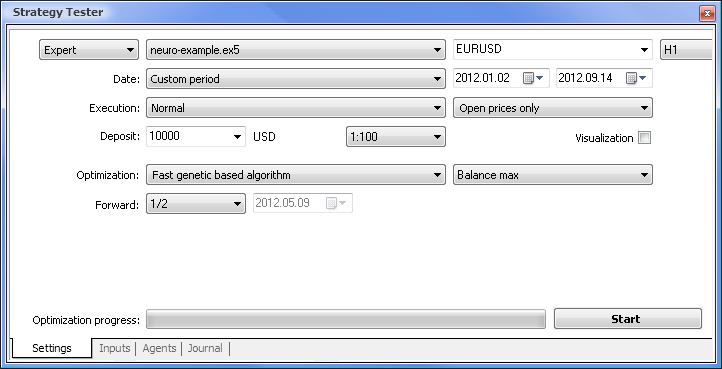• Date（日期） - 具体而言从一年的开头开始。周期越长，曲线拟合出现得就越少，结果也越好。
• Execution（执行） - normal（常规），Opening prices only（仅开盘价）。在 Every tick（每一价格变动）模式中测试没有意义，因为了除了当前值以外，EA 仅采用指标的最后 10 个值。
• Optimization（优化）可设置为使用慢速完整算法运行。然而，基因优化将提供更快的结果，这种优化在评估某个算法时特别有用。如果结果令人满意，也可以使用慢速完整算法以获得更加精确的结果。
• Forward（前进） 1/2 及更多让您能够评估您的 EA 在下一次优化之前要用多才时间才能生成获得的结果。
• Time frame（时间框架）Currency pair（货币对）可以依据您的需要设置。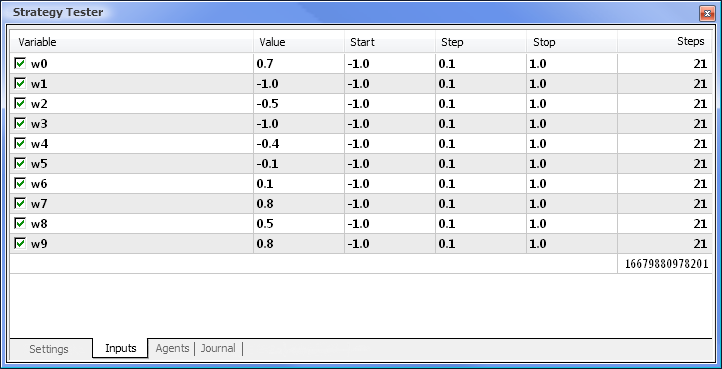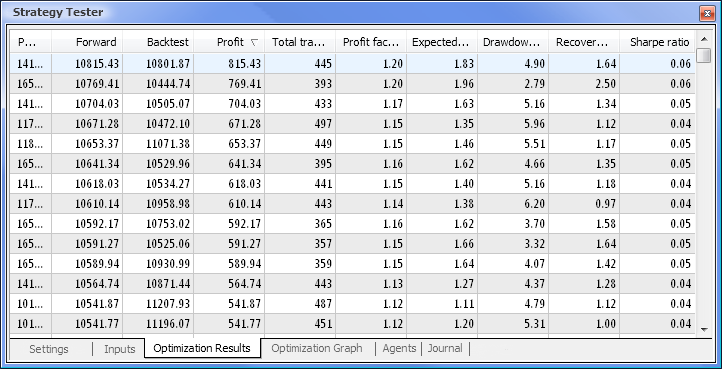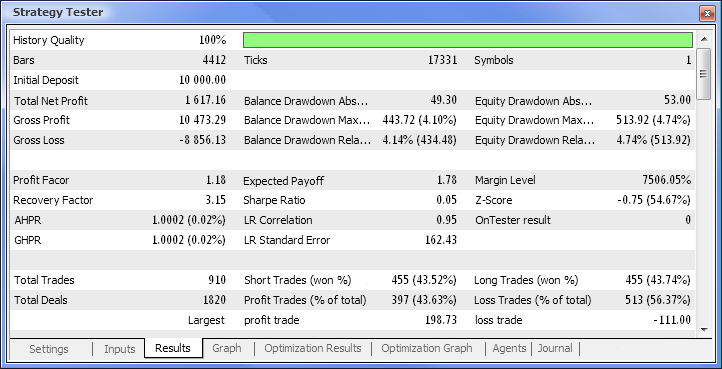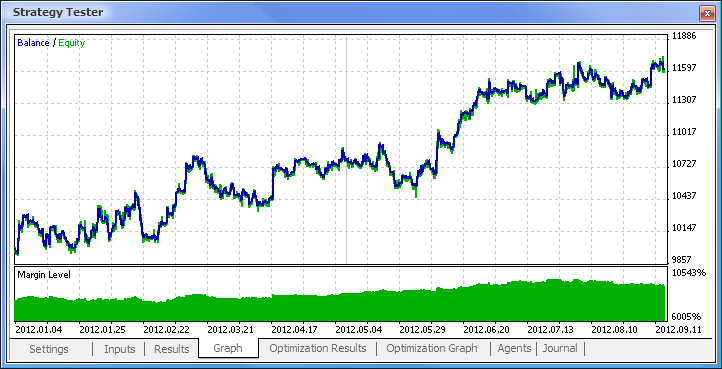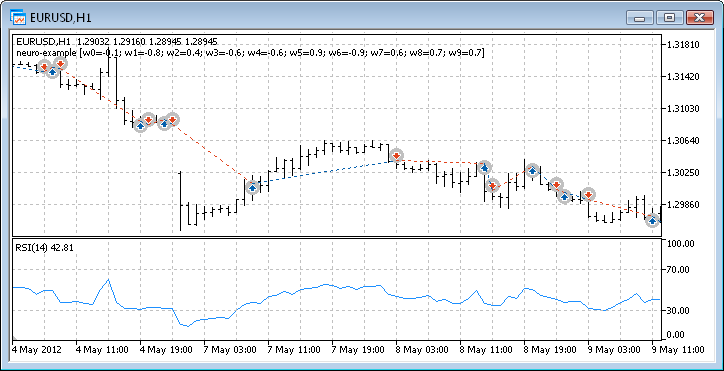### 神经网络的优点

• 快速移动平均线周期： 12;
• 慢速移动平均线周期： 26;
• 差异平均周期： 9;
• 价格类型：收盘价。

MACD 示例 MACD 神经网络示例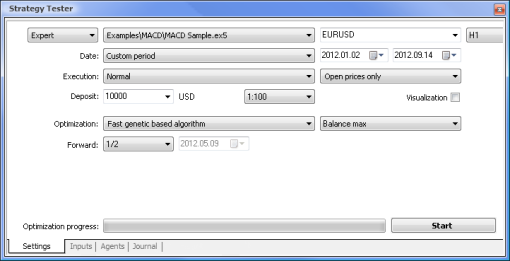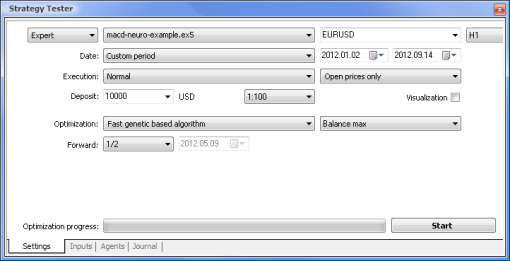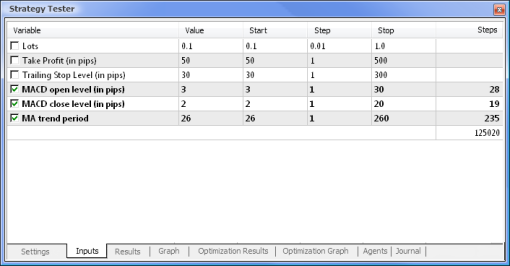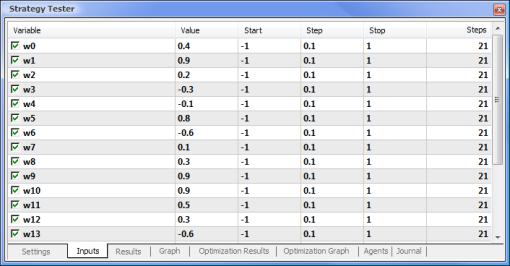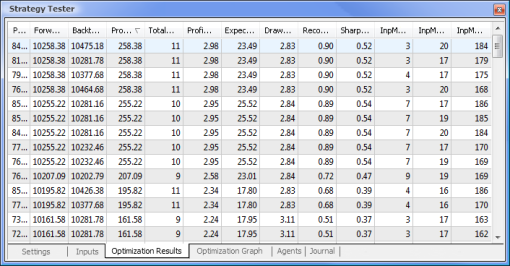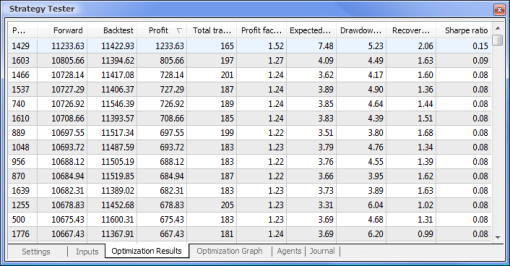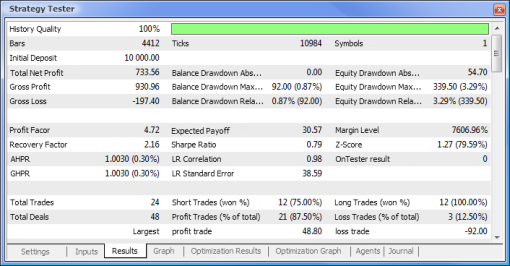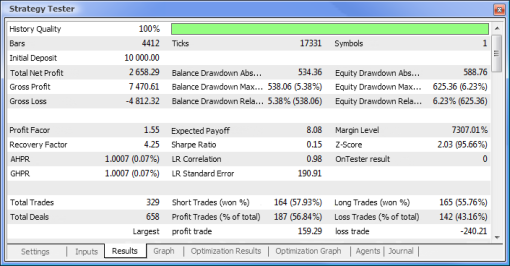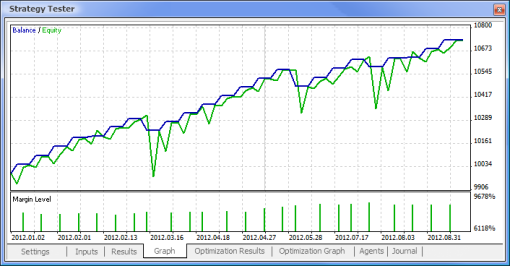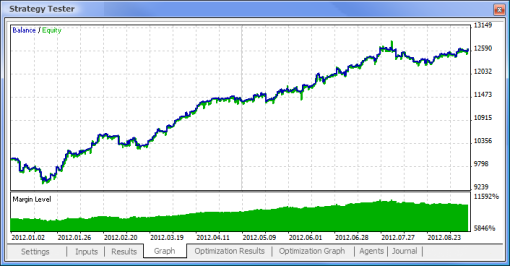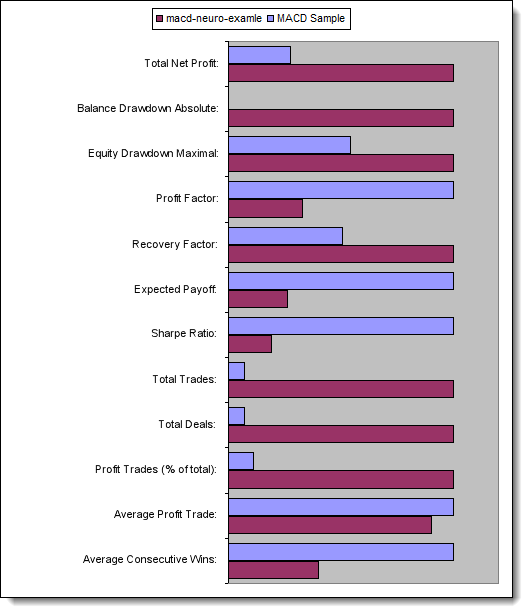### 总结

neuro-example.mq5 (7.45 KB)

#### 该作者的其他文章| 12 8月 2019 在 23:04| 26 8月 2019 在 09:23| 2 9月 2019 在 07:46
Hailong Song:

Thanks.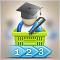如何从 MetaTrader 市场购买自动交易以及如何安装?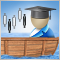统计套利交易策略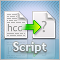如何准备 MetaTrader 5 报价用于其他应用程序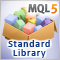探索标准库的交易策略类 - 自定义策略